# transprob

Estimate transition probabilities from credit ratings data

## Syntax

``[transMat,sampleTotals,idTotals] = transprob(data)``
``[transMat,sampleTotals,idTotals] = transprob(___,Name,Value)``

## Description

example

````[transMat,sampleTotals,idTotals] = transprob(data)` constructs a transition matrix from historical data of credit ratings.```

example

````[transMat,sampleTotals,idTotals] = transprob(___,Name,Value)` adds optional name-value pair arguments. ```

## Examples

collapse all

Using the historical credit rating table as input data from `Data_TransProb.mat` display the first ten rows and compute the transition matrix:

```load Data_TransProb data(1:10,:)```
```ans=10×3 table ID Date Rating ____________ _______________ _______ {'00010283'} {'10-Nov-1984'} {'CCC'} {'00010283'} {'12-May-1986'} {'B' } {'00010283'} {'29-Jun-1988'} {'CCC'} {'00010283'} {'12-Dec-1991'} {'D' } {'00013326'} {'09-Feb-1985'} {'A' } {'00013326'} {'24-Feb-1994'} {'AA' } {'00013326'} {'10-Nov-2000'} {'BBB'} {'00014413'} {'23-Dec-1982'} {'B' } {'00014413'} {'20-Apr-1988'} {'BB' } {'00014413'} {'16-Jan-1998'} {'B' } ```
```% Estimate transition probabilities with default settings transMat = transprob(data)```
```transMat = 8×8 93.1170 5.8428 0.8232 0.1763 0.0376 0.0012 0.0001 0.0017 1.6166 93.1518 4.3632 0.6602 0.1626 0.0055 0.0004 0.0396 0.1237 2.9003 92.2197 4.0756 0.5365 0.0661 0.0028 0.0753 0.0236 0.2312 5.0059 90.1846 3.7979 0.4733 0.0642 0.2193 0.0216 0.1134 0.6357 5.7960 88.9866 3.4497 0.2919 0.7050 0.0010 0.0062 0.1081 0.8697 7.3366 86.7215 2.5169 2.4399 0.0002 0.0011 0.0120 0.2582 1.4294 4.2898 81.2927 12.7167 0 0 0 0 0 0 0 100.0000 ```

Using the historical credit rating table input data from `Data_TransProb.mat`, compute the transition matrix using the `cohort` algorithm:

```%Estimate transition probabilities with 'cohort' algorithm transMatCoh = transprob(data,'algorithm','cohort')```
```transMatCoh = 8×8 93.1345 5.9335 0.7456 0.1553 0.0311 0 0 0 1.7359 92.9198 4.5446 0.6046 0.1560 0 0 0.0390 0.1268 2.9716 91.9913 4.3124 0.4711 0.0544 0 0.0725 0.0210 0.3785 5.0683 89.7792 4.0379 0.4627 0.0421 0.2103 0.0221 0.1105 0.6851 6.2320 88.3757 3.6464 0.2873 0.6409 0 0 0.0761 0.7230 7.9909 86.1872 2.7397 2.2831 0 0 0 0.3094 1.8561 4.5630 80.8971 12.3743 0 0 0 0 0 0 0 100.0000 ```

Using the historical credit rating data with ratings investment grade (`'IG'`), speculative grade (`'SG'`), and default (`'D'`), from `Data_TransProb.mat` display the first ten rows and compute the transition matrix:

`dataIGSG(1:10,:)`
```ans=10×3 table ID Date Rating ____________ _______________ ______ {'00011253'} {'04-Apr-1983'} {'IG'} {'00012751'} {'17-Feb-1985'} {'SG'} {'00012751'} {'19-May-1986'} {'D' } {'00014690'} {'17-Jan-1983'} {'IG'} {'00012144'} {'21-Nov-1984'} {'IG'} {'00012144'} {'25-Mar-1992'} {'SG'} {'00012144'} {'07-May-1994'} {'IG'} {'00012144'} {'23-Jan-2000'} {'SG'} {'00012144'} {'20-Aug-2001'} {'IG'} {'00012937'} {'07-Feb-1984'} {'IG'} ```
`transMatIGSG = transprob(dataIGSG,'labels',{'IG','SG','D'})`
```transMatIGSG = 3×3 98.6719 1.2020 0.1261 3.5781 93.3318 3.0901 0 0 100.0000 ```

Using the historical credit rating data with numeric ratings for investment grade (`1`), speculative grade (`2`), and default (`3`), from `Data_TransProb.mat` display the first ten rows and compute the transition matrix:

`dataIGSGnum(1:10,:)`
```ans=10×3 table ID Date Rating ____________ _______________ ______ {'00011253'} {'04-Apr-1983'} 1 {'00012751'} {'17-Feb-1985'} 2 {'00012751'} {'19-May-1986'} 3 {'00014690'} {'17-Jan-1983'} 1 {'00012144'} {'21-Nov-1984'} 1 {'00012144'} {'25-Mar-1992'} 2 {'00012144'} {'07-May-1994'} 1 {'00012144'} {'23-Jan-2000'} 2 {'00012144'} {'20-Aug-2001'} 1 {'00012937'} {'07-Feb-1984'} 1 ```
`transMatIGSGnum = transprob(dataIGSGnum,'labels',{1,2,3})`
```transMatIGSGnum = 3×3 98.6719 1.2020 0.1261 3.5781 93.3318 3.0901 0 0 100.0000 ```

Using a MATLAB® table containing the historical credit rating cell array input data (`dataCellFormat`) from `Data_TransProb.mat`, estimate the transition probabilities with default settings.

```load Data_TransProb transMat = transprob(dataCellFormat)```
```transMat = 8×8 93.1170 5.8428 0.8232 0.1763 0.0376 0.0012 0.0001 0.0017 1.6166 93.1518 4.3632 0.6602 0.1626 0.0055 0.0004 0.0396 0.1237 2.9003 92.2197 4.0756 0.5365 0.0661 0.0028 0.0753 0.0236 0.2312 5.0059 90.1846 3.7979 0.4733 0.0642 0.2193 0.0216 0.1134 0.6357 5.7960 88.9866 3.4497 0.2919 0.7050 0.0010 0.0062 0.1081 0.8697 7.3366 86.7215 2.5169 2.4399 0.0002 0.0011 0.0120 0.2582 1.4294 4.2898 81.2927 12.7167 0 0 0 0 0 0 0 100.0000 ```

Using the historical credit rating cell array input data (`dataCellFormat`), compute the transition matrix using the `cohort` algorithm:

```%Estimate transition probabilities with 'cohort' algorithm transMatCoh = transprob(dataCellFormat,'algorithm','cohort')```
```transMatCoh = 8×8 93.1345 5.9335 0.7456 0.1553 0.0311 0 0 0 1.7359 92.9198 4.5446 0.6046 0.1560 0 0 0.0390 0.1268 2.9716 91.9913 4.3124 0.4711 0.0544 0 0.0725 0.0210 0.3785 5.0683 89.7792 4.0379 0.4627 0.0421 0.2103 0.0221 0.1105 0.6851 6.2320 88.3757 3.6464 0.2873 0.6409 0 0 0.0761 0.7230 7.9909 86.1872 2.7397 2.2831 0 0 0 0.3094 1.8561 4.5630 80.8971 12.3743 0 0 0 0 0 0 0 100.0000 ```

This example shows how to visualize credit rating transitions that are used as an input to the `transprob` function. The example also describes how the `transprob` function treats rating transitions when the company data starts after the start date of the analysis, or when the end date of the analysis is after the last transition observed.

Sample Data

Set up fictitious sample data for illustration purposes.

```data = {'ABC','17-Feb-2015','AA'; 'ABC','6-Jul-2017','A'; 'LMN','12-Aug-2014','B'; 'LMN','9-Nov-2015','CCC'; 'LMN','7-Sep-2016','D'; 'XYZ','14-May-2013','BB'; 'XYZ','21-Jun-2016','BBB'}; data = cell2table(data,'VariableNames',{'ID','Date','Rating'}); disp(data)```
``` ID Date Rating _______ _______________ _______ {'ABC'} {'17-Feb-2015'} {'AA' } {'ABC'} {'6-Jul-2017' } {'A' } {'LMN'} {'12-Aug-2014'} {'B' } {'LMN'} {'9-Nov-2015' } {'CCC'} {'LMN'} {'7-Sep-2016' } {'D' } {'XYZ'} {'14-May-2013'} {'BB' } {'XYZ'} {'21-Jun-2016'} {'BBB'} ```

The `transprob` function understands that this panel-data format indicates the dates when a new rating is assigned to a given company. `transprob` assumes that such ratings remain unchanged, unless a subsequent row explicitly indicates a rating change. For example, for company `'ABC'`, `transprob` understands that the `'A'` rating is unchanged for any date after `'6-Jul-2017'` (indefinitely).

Compute Transition Matrix and Transition Counts

The `transprob` function returns a transition probability matrix as the primary output. There are also optional outputs that contain additional information for how many transitions occurred. For more information, see `transprob` for information on the optional outputs for both the `'cohort'` and the `'duration'` methods.

For illustration purposes, this example allows you to pick the `StartYear` (limited to `2014` or `2015` for this example) and the `EndYear` (`2016` or `2017`). This example also uses the `hDisplayTransitions` helper function (see the Local Functions section) to format the transitions information for ease of reading.

```StartYear =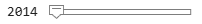2014; EndYear =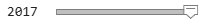2017; startDate = datetime(StartYear,12,31,'Locale','en_US'); endDate = datetime(EndYear,12,31,'Locale','en_US'); RatingLabels = ["AAA","AA","A","BBB","BB","B","CCC","D"]; [tm,st,it] = transprob(data,'startDate',startDate,'endDate',endDate,'algorithm','cohort','labels',RatingLabels);```

The transition probabilities of the `TransMat` output indicate the probability of migrating between ratings. The probabilities are expressed in %, that is, they are multiplied by 100.

`hDisplayTransitions(tm,RatingLabels,"Transition Matrix")`
```Transition Matrix AAA AA A BBB BB B CCC D ___ __ ___ ___ __ _ ___ ___ AAA 100 0 0 0 0 0 0 0 AA 0 50 50 0 0 0 0 0 A 0 0 100 0 0 0 0 0 BBB 0 0 0 100 0 0 0 0 BB 0 0 0 50 50 0 0 0 B 0 0 0 0 0 0 100 0 CCC 0 0 0 0 0 0 0 100 D 0 0 0 0 0 0 0 100 ```

The transition counts are stored in the `sampleTotals` optional output and indicate how many transitions occurred between ratings for the entire sample (that is, all companies).

`hDisplayTransitions(st.totalsMat,RatingLabels,"Transition counts, all companies")`
```Transition counts, all companies AAA AA A BBB BB B CCC D ___ __ _ ___ __ _ ___ _ AAA 0 0 0 0 0 0 0 0 AA 0 1 1 0 0 0 0 0 A 0 0 0 0 0 0 0 0 BBB 0 0 0 1 0 0 0 0 BB 0 0 0 1 1 0 0 0 B 0 0 0 0 0 0 1 0 CCC 0 0 0 0 0 0 0 1 D 0 0 0 0 0 0 0 1 ```

The third output of `transprob` is `idTotals` that contains information about transitions at an ID level, company by company (in the same order that the companies appear in the input data).

Select a company to display the transition counts and a corresponding visualization of the transitions. The `hPlotTransitions` helper function (see the Local Functions section) shows the transitions history for a company.

```CompanyID =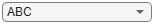"ABC"; UniqueIDs = unique(data.ID,'stable'); [~,CompanyIndex] = ismember(CompanyID,UniqueIDs); hDisplayTransitions(it(CompanyIndex).totalsMat,RatingLabels,strcat("Transition counts, company ID: ",CompanyID))```
```Transition counts, company ID: ABC AAA AA A BBB BB B CCC D ___ __ _ ___ __ _ ___ _ AAA 0 0 0 0 0 0 0 0 AA 0 1 1 0 0 0 0 0 A 0 0 0 0 0 0 0 0 BBB 0 0 0 0 0 0 0 0 BB 0 0 0 0 0 0 0 0 B 0 0 0 0 0 0 0 0 CCC 0 0 0 0 0 0 0 0 D 0 0 0 0 0 0 0 0 ```
`hPlotTransitions(CompanyID,startDate,endDate,data,RatingLabels)`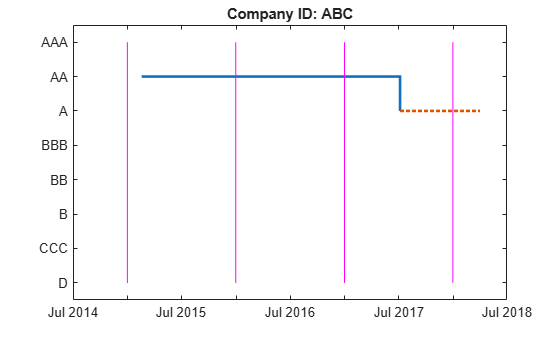To understand how `transprob` handles data when the first observed date is after the start date of the analysis, or whose last observed date occurs before the end date of the analysis, consider the following example. For company `'ABC'` suppose that the analysis has a start date of `31-Dec-2014` and end date of `31-Dec-2017`. There are only two transitions reported for this company for that analysis time window. The first observation for `'ABC'` happened on `17-Feb-2015`. So the `31-Dec-2015` snapshot is the first time the company is observed. By `31-Dec-2016`, the company remained in the original `'AA'` rating. By `31-Dec-2017`, a downgrade to `'A'` is recorded. Consistent with this, the transition counts show one transition from `'AA'` to `'AA'` (from the end of 2015 to the end of 2016), and one transition from `'AA'` to `'A'` (from the end of 2016 to the end of 2017). The plot shows the last rating as a dotted red line to emphasize that the last rating in the data is extrapolated indefinitely into the future. There is no extrapolation into the past; the company's history is ignored until a company rating is known for an entire transition period (`31-Dec-2015` through `31-Dec-2016` in the case of `'ABC'`).

Compute Transition Matrix Containing `NR` (Not Rated) Rating

Consider a different sample data containing only a single company `'DEF'`. The data contains transitions of company `'DEF'` from `'A'` to `'NR'` rating and a subsequent transition from `'NR'` to `'BBB'`.

```dataNR = {'DEF','17-Mar-2011','A'; 'DEF','24-Mar-2014','NR'; 'DEF','26-Sep-2016','BBB'}; dataNR = cell2table(dataNR,'VariableNames',{'ID','Date','Rating'}); disp(dataNR)```
``` ID Date Rating _______ _______________ _______ {'DEF'} {'17-Mar-2011'} {'A' } {'DEF'} {'24-Mar-2014'} {'NR' } {'DEF'} {'26-Sep-2016'} {'BBB'} ```

`transprob` treats `'NR'` as another rating. The transition matrix below shows the estimated probability of transitioning into and out of `'NR'`.

```StartYearNR = 2010; EndYearNR = 2018; startDateNR = datetime(StartYearNR,12,31,'Locale','en_US'); endDateNR = datetime(EndYearNR,12,31,'Locale','en_US'); CompanyID_NR = "DEF"; RatingLabelsNR = ["AAA","AA","A","BBB","BB","B","CCC","D","NR"]; [tmNR,~,itNR] = transprob(dataNR,'startDate',startDateNR,'endDate',endDateNR,'algorithm','cohort','labels',RatingLabelsNR); hDisplayTransitions(tmNR,RatingLabelsNR,"Transition Matrix")```
```Transition Matrix AAA AA A BBB BB B CCC D NR ___ ___ ______ ___ ___ ___ ___ ___ ______ AAA 100 0 0 0 0 0 0 0 0 AA 0 100 0 0 0 0 0 0 0 A 0 0 66.667 0 0 0 0 0 33.333 BBB 0 0 0 100 0 0 0 0 0 BB 0 0 0 0 100 0 0 0 0 B 0 0 0 0 0 100 0 0 0 CCC 0 0 0 0 0 0 100 0 0 D 0 0 0 0 0 0 0 100 0 NR 0 0 0 50 0 0 0 0 50 ```

Display the transition counts and corresponding visualization of the transitions.

`hDisplayTransitions(itNR.totalsMat,RatingLabelsNR,strcat("Transition counts, company ID: ",CompanyID_NR))`
```Transition counts, company ID: DEF AAA AA A BBB BB B CCC D NR ___ __ _ ___ __ _ ___ _ __ AAA 0 0 0 0 0 0 0 0 0 AA 0 0 0 0 0 0 0 0 0 A 0 0 2 0 0 0 0 0 1 BBB 0 0 0 2 0 0 0 0 0 BB 0 0 0 0 0 0 0 0 0 B 0 0 0 0 0 0 0 0 0 CCC 0 0 0 0 0 0 0 0 0 D 0 0 0 0 0 0 0 0 0 NR 0 0 0 1 0 0 0 0 1 ```
`hPlotTransitions(CompanyID_NR,startDateNR,endDateNR,dataNR,RatingLabelsNR)`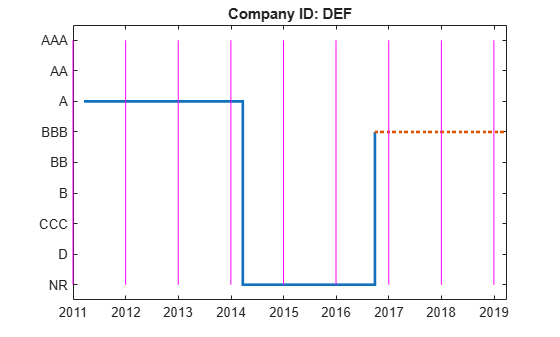To remove the `'NR'` from the transition matrix, use the `'excludeLabels'` name-value input argument in `transprob`. The list of labels to exclude may or may not be specified in the name-value pair argument `labels`. For example, both `RatingLabels` and `RatingLabelsNR` generate the same output from `transprob`.

```[tmNR,stNR,itNR] = transprob(dataNR,'startDate',startDateNR,'endDate',endDateNR,'algorithm','cohort','labels',RatingLabelsNR,'excludeLabels','NR'); hDisplayTransitions(tmNR,RatingLabels,"Transition Matrix")```
```Transition Matrix AAA AA A BBB BB B CCC D ___ ___ ___ ___ ___ ___ ___ ___ AAA 100 0 0 0 0 0 0 0 AA 0 100 0 0 0 0 0 0 A 0 0 100 0 0 0 0 0 BBB 0 0 0 100 0 0 0 0 BB 0 0 0 0 100 0 0 0 B 0 0 0 0 0 100 0 0 CCC 0 0 0 0 0 0 100 0 D 0 0 0 0 0 0 0 100 ```

Display the transition counts and corresponding visualization of the transitions.

`hDisplayTransitions(itNR.totalsMat,RatingLabels,strcat("Transition counts, company ID: ",CompanyID_NR))`
```Transition counts, company ID: DEF AAA AA A BBB BB B CCC D ___ __ _ ___ __ _ ___ _ AAA 0 0 0 0 0 0 0 0 AA 0 0 0 0 0 0 0 0 A 0 0 2 0 0 0 0 0 BBB 0 0 0 2 0 0 0 0 BB 0 0 0 0 0 0 0 0 B 0 0 0 0 0 0 0 0 CCC 0 0 0 0 0 0 0 0 D 0 0 0 0 0 0 0 0 ```
`hPlotTransitions(CompanyID_NR,startDateNR,endDateNR,dataNR,RatingLabels)`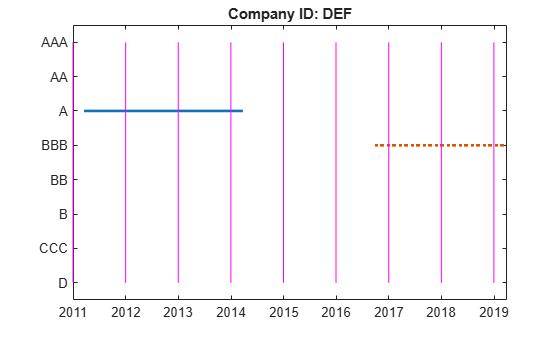Consistent with the previous plot, the transition counts still show two transitions from `'A'` to `'A'` (from the end of 2012 to the end of 2014), and two transitions from `'BBB'` to `'BBB'` (from the end of 2017 to the end of 2019).

However, different from the previous plot, specifying `'NR'` using the `'excludeLabels'` name-value input argument of `transprob` removes any transitions into and out of the `'NR'` rating.

Local Functions

```function hDisplayTransitions(TransitionsData,RatingLabels,Title) % Helper function to format transition information outputs TransitionsAsTable = array2table(TransitionsData,... 'VariableNames',RatingLabels,'RowNames',RatingLabels); fprintf('\n%s\n\n',Title) disp(TransitionsAsTable) end function hPlotTransitions(CompanyID,startDate,endDate,data,RatingLabels) % Helper function to visualize transitions between ratings Ind = string(data.ID)==CompanyID; DatesOriginal = datetime(data.Date(Ind),'Locale','en_US'); RatingsOriginal = categorical(data.Rating(Ind),flipud(RatingLabels(:)),flipud(RatingLabels(:))); stairs(DatesOriginal,RatingsOriginal,'LineWidth',2) hold on; % Indicate rating extrapolated into the future (arbitrarily select 91 % days after endDate as the last date on the plot) endDateExtrap = endDate+91; if endDateExtrap>DatesOriginal(end) DatesExtrap = [DatesOriginal(end); endDateExtrap]; RatingsExtrap = [RatingsOriginal(end); RatingsOriginal(end)]; stairs(DatesExtrap,RatingsExtrap,'LineWidth',2,'LineStyle',':') end hold off; % Add lines to indicate the snapshot dates % transprob uses 1 as the default for 'snapsPerYear', hardcoded here for simplicity % The call to cfdates generates the exact same snapshot dates that transprob uses snapsPerYear = 1; snapDates = cfdates(startDate-1,endDate,snapsPerYear)'; yLimits = ylim; for ii=1:length(snapDates) line([snapDates(ii) snapDates(ii)],yLimits,'Color','m') end title(strcat("Company ID: ",CompanyID)) end```

## Input Arguments

collapse all

Using `transprob` to estimate transition probabilities given credit ratings historical data (that is, credit migration data), the `data` input can be one of the following:

• An `nRecords`-by-`3` MATLAB® table containing the historical credit ratings data of the form:

``` ID Date Rating __________ _____________ ______ '00010283' '10-Nov-1984' 'CCC' '00010283' '12-May-1986' 'B' '00010283' '29-Jun-1988' 'CCC' '00010283' '12-Dec-1991' 'D' '00013326' '09-Feb-1985' 'A' '00013326' '24-Feb-1994' 'AA' '00013326' '10-Nov-2000' 'BBB' '00014413' '23-Dec-1982' 'B'```
where each row contains an ID (column 1), a date (column 2), and a credit rating (column 3). Column 3 is the rating assigned to the corresponding ID on the corresponding date. All information corresponding to the same ID must be stored in contiguous rows. Sorting this information by date is not required, but recommended for efficiency. When using a MATLAB table input, the names of the columns are irrelevant, but the ID, date and rating information are assumed to be in the first, second, and third columns, respectively. Also, when using a table input, the first and third columns can be categorical arrays, and the second can be a datetime array. The following summarizes the supported data types for table input:

Data Input TypeID (1st Column)Date (2nd Column)Rating (3rd Column)
Table

• Numeric array

• Cell array of character vectors

• Categorical array

• Numeric array

• Cell array of character vectors

• Datetime array

• Numeric array

• Cell array of character vectors

• Categorical array

• An `nRecords`-by-`3` cell array of character vectors containing the historical credit ratings data of the form:

```'00010283' '10-Nov-1984' 'CCC' '00010283' '12-May-1986' 'B' '00010283' '29-Jun-1988' 'CCC' '00010283' '12-Dec-1991' 'D' '00013326' '09-Feb-1985' 'A' '00013326' '24-Feb-1994' 'AA' '00013326' '10-Nov-2000' 'BBB' '00014413' '23-Dec-1982' 'B'```
where each row contains an ID (column 1), a date (column 2), and a credit rating (column 3). Column 3 is the rating assigned to the corresponding ID on the corresponding date. All information corresponding to the same ID must be stored in contiguous rows. Sorting this information by date is not required, but recommended for efficiency. IDs, dates, and ratings are stored in character vector format, but they can also be entered in numeric format. The following summarizes the supported data types for cell array input:

Data Input TypeID (1st Column)Date (2nd Column)Rating (3rd Column)
Cell

• Numeric elements

• Character vector elements

• Numeric elements

• Character vector elements

• Numeric elements

• Character vector elements

• A preprocessed data structure obtained using `transprobprep`. This data structure contains the fields`'idStart'`, `'numericDates'`, `'numericRatings'`, and `'ratingsLabels'`.

Data Types: `table` | `cell` | `struct`

### Name-Value Arguments

Specify optional pairs of arguments as `Name1=Value1,...,NameN=ValueN`, where `Name` is the argument name and `Value` is the corresponding value. Name-value arguments must appear after other arguments, but the order of the pairs does not matter.

Before R2021a, use commas to separate each name and value, and enclose `Name` in quotes.

Example: ```transMat = transprob(data,'algorithm','cohort')```

Estimation algorithm, specified as the comma-separated pair consisting of `'algorithm'` and a character vector with a value of `'duration'` or `'cohort'`.

Data Types: `char`

End date of the estimation time window, specified as the comma-separated pair consisting of `'endDate'` and a scalar datetime, string, date character vector, or serial date number. The `endDate` cannot be a date before the `startDate`.

Data Types: `char` | `double` | `string` | `datetime`

Credit-rating scale, specified as the comma-separated pair consisting of `'labels'` and a `nRatings`-by-`1`, or `1`-by-`nRatings` cell array of character vectors.

`labels` must be consistent with the ratings labels used in the third column of `data`. Use a cell array of numbers for numeric ratings, and a cell array for character vectors for categorical ratings.

Note

When the input argument `data` is a preprocessed data structure obtained from a previous call to `transprobprep`, this optional input for `'labels` is unused because the labels in the `'ratingsLabels'` field of `transprobprep` take priority.

Data Types: `cell`

Number of credit-rating snapshots per year to be considered for the estimation, specified as the comma-separated pair consisting of `'snapsPerYear'` and a numeric value of `1`, `2`, `3`, `4`, `6`, or `12`.

Note

This parameter is only used with the `'cohort'` `algorithm`.

Data Types: `double`

Start date of the estimation time window, specified as the comma-separated pair consisting of `'startDate'` and a scalar datetime, string, date character vector, or serial date number.

Data Types: `char` | `double` | `string` | `datetime`

Length of the transition interval, in years, specified as the comma-separated pair consisting of `'transInterval'` and a numeric value.

Data Types: `double`

Label that is excluded from the transition probability computation, specified as the comma-separated pair consisting of `'excludeLabels'` and a character vector, string, or numerical rating.

If multiple labels are to be excluded, `'excludeLabels'` must be a cell array containing all of the labels for exclusion. The type of the labels given in `'excludeLabels'` must be consistent with the data type specified in the `labels` input.

The list of labels to exclude may or may not be specified in `labels`.

Data Types: `double` | `char` | `string`

## Output Arguments

collapse all

Matrix of transition probabilities in percent, returned as a `nRatings`-by-`nRatings` transition matrix.

Structure with sample totals, returned with fields:

• `totalsVec` — A vector of size `1`-by-`nRatings`.

• `totalsMat` — A matrix of size `nRatings`-by-`nRatings`.

• `algorithm` — A character vector with values `'duration'` or `'cohort'`.

For the `'duration'` algorithm, `totalsMat`(i,j) contains the total transitions observed out of rating i into ratingj (all the diagonal elements are zero). The total time spent on rating i is stored in `totalsVec`(i). For example, if there are three rating categories, Investment Grade (`IG`), Speculative Grade (`SG`), and Default (`D`), and the following information:

```Total time spent IG SG D in rating: 4859.09 1503.36 1162.05 Transitions IG SG D out of (row) IG 0 89 7 into (column): SG 202 0 32 D 0 0 0```
Then
```totals.totalsVec = [4859.09 1503.36 1162.05] totals.totalsMat = [ 0 89 7 202 0 32 0 0 0] totals.algorithm = 'duration'```

For the `'cohort'` algorithm, `totalsMat`(i,j) contains the total transitions observed from rating i to rating j, and `totalsVec`(i) is the initial count in rating i. For example, given the following information:

```Initial count IG SG D in rating: 4808 1572 1145 Transitions IG SG D from (row) IG 4721 80 7 to (column): SG 193 1347 32 D 0 0 1145```
Then

```totals.totalsVec = [4808 1572 1145] totals.totalsMat = [4721 80 7 193 1347 32 0 0 1145 totals.algorithm = 'cohort'```

IDs totals, returned as a struct array of size `nIDs`-by-`1`, where nIDs is the number of distinct IDs in column 1 of `data` when this is a table or cell array or, equivalently, equal to the length of the `idStart` field minus 1 when `data` is a preprocessed data structure from `transprobprep`. For each ID in the sample, `idTotals` contains one structure with the following fields:

• `totalsVec` — A sparse vector of size `1`-by-`nRatings`.

• `totalsMat` — A sparse matrix of size `nRatings`-by-`nRatings`.

• `algorithm` — A character vector with values `'duration'` or `'cohort'`.

These fields contain the same information described for the output `sampleTotals`, but at an ID level. For example, for `'duration'`, `idTotals`(k).`totalsVec` contains the total time that the k-th company spent on each rating.

collapse all

### Cohort Estimation

The cohort algorithm estimates the transition probabilities based on a sequence of snapshots of credit ratings at regularly spaced points in time.

If the credit rating of a company changes twice between two snapshot dates, the intermediate rating is overlooked and only the initial and final ratings influence the estimates.

### Duration Estimation

Unlike the cohort method, the duration algorithm estimates the transition probabilities based on the full credit ratings history, looking at the exact dates on which the credit rating migrations occur.

There is no concept of snapshots in this method, and all credit rating migrations influence the estimates, even when a company's rating changes twice within a short time.

## Algorithms

collapse all

### Cohort Estimation

The algorithm first determines a sequence t0,...,tK of snapshot dates. The elapsed time, in years, between two consecutive snapshot dates tk-1 and tk is equal to `1` / ns, where ns is the number of snapshots per year. These K +`1` dates determine K transition periods.

The algorithm computes ${N}_{i}^{n}$, the number of transition periods in which obligor n starts at rating i. These are added up over all obligors to get Ni, the number of obligors in the sample that start a period at rating i. The number periods in which obligor n starts at rating i and ends at rating j, or migrates from i to j, denoted by${N}_{ij}^{n}$, is also computed. These are also added up to get ${N}_{ij}^{}$, the total number of migrations from i to j in the sample.

The estimate of the transition probability from i to j in one period, denoted by${P}_{ij}^{}$, is

`${P}_{ij}^{}=\frac{Nij}{Ni}$`

These probabilities are arranged in a one-period transition matrix P0, where the i,j entry in P0 is Pij.

If the number of snapshots per year ns is 4 (quarterly snapshots), the probabilities in P0 are 3-month (or 0.25-year) transition probabilities. You may, however, be interested in 1-year or 2-year transition probabilities. The latter time interval is called the transition interval, Δt, and it is used to convert P0 into the final transition matrix, P, according to the formula:

`$P={P}_{0}^{ns\ast △t}$`

For example, if ns = `4` and Δt = `2`, P contains the two-year transition probabilities estimated from quarterly snapshots.

Note

For the cohort algorithm, optional output arguments `idTotals` and `sampleTotals` from `transprob` contain the following information:

• `idTotals(n).totalsVec` = $\left({N}_{i}^{n}\right)\forall i$

• `idTotals(n).totalsMat` = $\left({N}_{i,j}^{n}\right)\forall ij$

• `idTotals(n).algorithm` = `'cohort'`

• `sampleTotals.totalsVec` = $\left({N}_{i}^{}\right)\forall i$

• `sampleTotals.totalsMat` = $\left({N}_{i,j}^{}\right)\forall ij$

• `sampleTotals.algorithm` = `'cohort'`

For efficiency, the vectors and matrices in `idTotals` are stored as sparse arrays.

When ratings must be excluded (see the `excludeLabels` name-value input argument), all transitions involving the excluded ratings are removed from the sample. For example, if the `‘NR’` rating must be excluded, any transitions into `‘NR’` and out of `‘NR’` are excluded from the sample. The total counts for all other ratings are adjusted accordingly. For more information, see Visualize Transitions Data for transprob.

### Duration Estimation

The algorithm computes ${T}_{i}^{n}$, the total time that obligor n spends in rating i within the estimation time window. These quantities are added up over all obligors to get ${T}_{i}^{}$, the total time spent in rating i, collectively, by all obligors in the sample. The algorithm also computes ${T}_{ij}^{n}$, the number times that obligor n migrates from rating i to rating j, with i not equal to j, within the estimation time window. And it also adds them up to get ${T}_{ij}^{}$, the total number of migrations, by all obligors in the sample, from the rating i to j, with i not equal to j.

To estimate the transition probabilities, the duration algorithm first computes a generator matrix $\Lambda$. Each off-diagonal entry of this matrix is an estimate of the transition rate out of rating i into rating j, and is

`${\lambda }_{ij}^{}=\frac{{T}_{ij}^{}}{{T}_{i}^{}},i\ne j$`

The diagonal entries are computed as:

`${\lambda }_{ii}^{}=-\sum _{j\ne i}^{}{\lambda }_{ij}^{}$`

With the generator matrix and the transition interval Δt (e.g., Δt = `2` corresponds to two-year transition probabilities), the transition matrix is obtained as $P=\mathrm{exp}\left(\Delta t\Lambda \right)$, where exp denotes matrix exponentiation (`expm` in MATLAB).

Note

For the duration algorithm, optional output arguments `idTotals` and `sampleTotals` from `transprob` contain the following information:

• `idTotals(n).totalsVec` = $\left({T}_{i}^{n}\right)\forall i$

• `idTotals(n).totalsMat` = $\left({T}_{i,j}^{n}\right)\forall ij$

• `idTotals(n).algorithm` = `'duration'`

• `sampleTotals.totalsVec` = $\left({T}_{i}^{}\right)\forall i$

• `sampleTotals.totalsMat` = $\left({T}_{i,j}^{}\right)\forall ij$

• `sampleTotals.algorithm` = `'duration'`

For efficiency, the vectors and matrices in `idTotals` are stored as sparse arrays.

When ratings must be excluded (see the `excludeLabels` name-value input argument), all transitions involving the exclude ratings are removed from the sample. For example, if the `‘NR’` rating must be excluded, any transitions into `‘NR’` and out of `‘NR’` are excluded from the sample. The total time spent in `‘NR’` (or any other excluded rating) is also removed.

 Hanson, S., T. Schuermann. "Confidence Intervals for Probabilities of Default." Journal of Banking & Finance. Vol. 30(8), Elsevier, August 2006, pp. 2281–2301.

 Löffler, G., P. N. Posch. Credit Risk Modeling Using Excel and VBA. West Sussex, England: Wiley Finance, 2007.

 Schuermann, T. "Credit Migration Matrices." in E. Melnick, B. Everitt (eds.), Encyclopedia of Quantitative Risk Analysis and Assessment. Wiley, 2008.